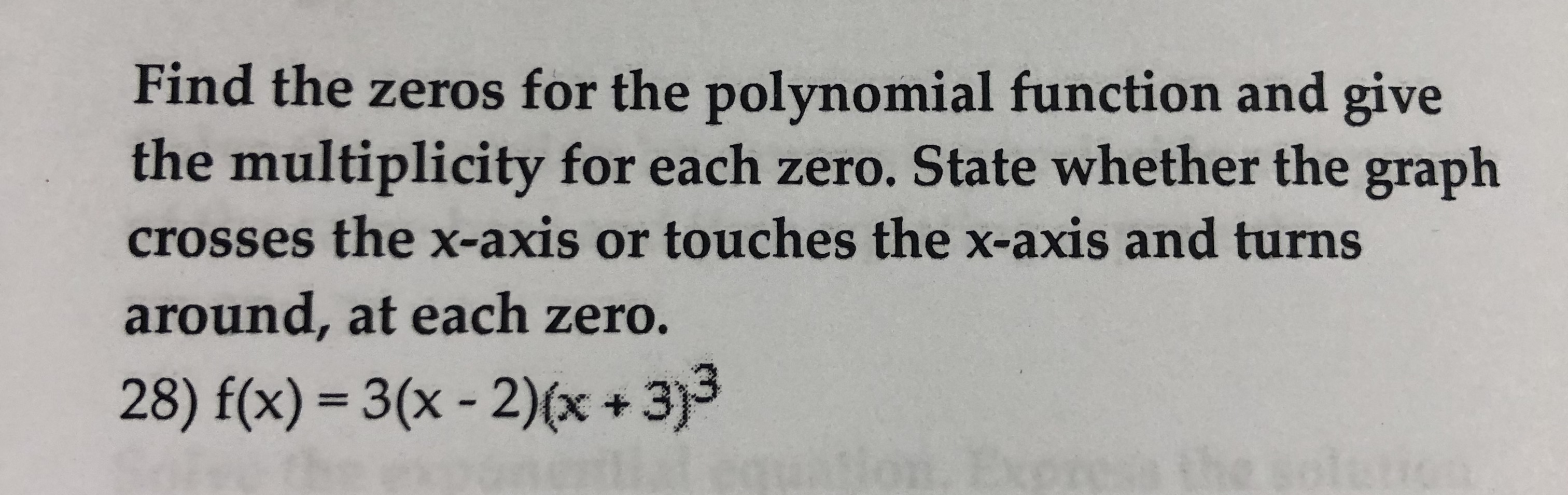Find the zeros for the polynomial function and givethe multiplicity for each zero. State whether the graphcrosses the x-axis or touches the x-axis and turnsaround, at each zero.28) f(x) 3(x-2)x+3)3

Questionhelp_outlineImage TranscriptioncloseFind the zeros for the polynomial function and give the multiplicity for each zero. State whether the graph crosses the x-axis or touches the x-axis and turns around, at each zero. 28) f(x) 3(x-2)x+3)3 fullscreen
Step 1

The polynomial function is given as

Step 2

Use f(x) = 0, and obtain the zeros and the...

Want to see the full answer?

See Solution

Want to see this answer and more?

Our solutions are written by experts, many with advanced degrees, and available 24/7

See Solution
Tagged in

Polynomials